# Free online courseFundamentals of Physics with Ramamurti Shankar

4.97

(36)

## 28h44m of online video course

Quality content and selected for your learning.

## Exercises to train your knowledge

Many of the courses available have exercises to help with learning.

This course is free today and always will be, from start to finish you will pay nothing.

## Physics

Prepare your knowledge in physics and study hard to pass the exams. Here we have free online classes for you to studyTeacher

##### Ramamurti Shankar
###### 2 Courses / 2622 students

Yales philosophy of teaching and learning begins with the aim of training a broadly based, highly disciplined intellect without specifying in advance how that intellect will be used. The Yale Courses channel provides entry into the core of the University--its classrooms and academic programs--including complete sets of lectures from the Open Yale Courses initiative.

## Course content preview

There are no comments yet for this course.Watch the 1st video of the course

## Course content1h13m

### 1. Course Introduction and Newtonian Mechanics

For more information about Professor Shankar's book based on the lectures from this course, Fundamentals of Physics: Mechanics, Relativity, and Thermodynamics, visit http://bit.ly/1jFIqNu.

Fundamentals of Physics (PHYS 200)

Professor Shankar introduces the course and answers student questions about the material and the requirements. He gives an overview of Newtonian mechanics and explains its two components: kinematics and dynamics. He then reviews basic concepts in calculus through two key equations: x = x0 + v0t + ½ at2 and v2 = v02+ 2 a (x-x0), tracing the fate of a particle in one dimension along the x-axis.

00:00 - Chapter 1. Introduction and Course Organization
21:25 - Chapter 2. Newtonian Mechanics: Dynamics and Kinematics
28:20 - Chapter 3. Average and Instantaneous Rate of Motion
37:56 - Chapter 4. Motion at Constant Acceleration
52:37 - Chapter 5. Example Problem: Physical Meaning of Equations
01:08:42 - Chapter 6. Derive New Relations Using Calculus Laws of Limits

Comple1h06m

### 2. Vectors in Multiple Dimensions

For more information about Professor Shankar's book based on the lectures from this course, Fundamentals of Physics: Mechanics, Relativity, and Thermodynamics, visit http://bit.ly/1jFIqNu.

Fundamentals of Physics (PHYS 200)

In this lecture, Professor Shankar discusses motion in more than one dimension. Vectors are introduced and discussed in multiple dimensions. Vector magnitude and direction are also explained. Null vectors, minus vectors, unit and velocity vectors are discussed along with their properties. Finally, several specific problems are solved to demonstrate how vectors can be added, and problems of projectile motion are expounded.

00:00 - Chapter 1. Review of Motion at Constant Acceleration
04:45 - Chapter 2. Vector Motion 2D Space: Properties
22:57 - Chapter 3. Choice of Basis Axis and Vector Transformation
38:20 - Chapter 4. Velocity Vectors: Derivatives of Displacement Vectors
43:40 - Chapter 5. Derivatives of Vectors: Application to Circular Motion
54:00 - Chapter 6.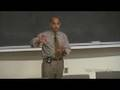1h08m

### 3. Newton's Laws of Motion

For more information about Professor Shankar's book based on the lectures from this course, Fundamentals of Physics: Mechanics, Relativity, and Thermodynamics, visit http://bit.ly/1jFIqNu.

Fundamentals of Physics (PHYS 200)

This lecture introduces Newton's Laws of Motion. The First Law on inertia states that every object will remain in a state of rest or uniform motion in a straight line unless acted upon by an external force. The Second Law (F = ma) relates the cause (the force F) to the acceleration. Several different forces are discussed in the context of this law. The lecture ends with the Third Law which states that action and reaction are equal and opposite.

00:00 - Chapter 1. Review of Vectors
09:29 - Chapter 2. Introduction to Newton's Laws of Motion, 1st Law and Inertial Frames
19:51 - Chapter 3. Second Law and Measurements as conventions
37:13 - Chapter 4. Nature of Forces and Their Relationship to Second Law
50:50 - Chapter 5. Newton's Third Law
01:03:15 - Chapter 6. Weig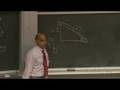1h07m

### 4. Newton's Laws (cont.) and Inclined Planes

For more information about Professor Shankar's book based on the lectures from this course, Fundamentals of Physics: Mechanics, Relativity, and Thermodynamics, visit http://bit.ly/1jFIqNu.

Fundamentals of Physics (PHYS 200)

The lecture begins with the application of Newton's three laws, with the warning that they are not valid for objects that move at speeds comparable to the speed of light or objects that are incredibly small and of the atomic scale. Friction and static friction are discussed. The dreaded inclined plane is dealt with head on. Finally, Professor Shankar explains the motion of objects using Newton's laws in specific problems related to objects in circular motion, such as roller coasters and a planet orbiting the Sun.

00:00 - Chapter 1. Continuation of Types of External Forces
14:50 - Chapter 2. Kinetic and Static Friction
31:34 - Chapter 3. Inclined Planes
49:04 - Chapter 4. Pulleys
57:30 - Chapter 5. Friction and Circular Motion: Roundabouts, Loop-the-Loop

Complete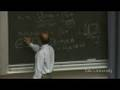1h10m

### 5. Work-Energy Theorem and Law of Conservation of Energy

For more information about Professor Shankar's book based on the lectures from this course, Fundamentals of Physics: Mechanics, Relativity, and Thermodynamics, visit http://bit.ly/1jFIqNu.

Fundamentals of Physics (PHYS 200)

The lecture begins with a review of the loop-the-loop problem. Professor Shankar then reviews basic terminology in relation to work, kinetic energy and potential energy. He then goes on to define the Work-Energy Theorem. Finally, the Law of Conservation of Energy is discussed and demonstrated with specific examples.

00:00 - Chapter 1. More on Loop-the-Loop and Intro to Concept of Energy
11:57 - Chapter 2. Work-Energy Theorem and Power
29:19 - Chapter 3. Conservation of Energy: K2 + U2 = K1 + U1
44:39 - Chapter 4. Friction Force Effect on Work-Energy Theorem
56:13 - Chapter 5. Calculus Review: Small Changes

Complete course materials are available at the Yale Online website: online.yale.edu

This course was recorded in Fall 2006.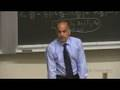1h11m

### 6. Law of Conservation of Energy in Higher Dimensions

For more information about Professor Shankar's book based on the lectures from this course, Fundamentals of Physics: Mechanics, Relativity, and Thermodynamics, visit http://bit.ly/1jFIqNu.

Fundamentals of Physics (PHYS 200)

The discussion on the Law of Conservation of Energy continues but is applied in higher dimensions. The notion of a function with two variables is reviewed. Conservative forces are explained and students are taught how to recognize and manufacture them.

00:00 - Chapter 1. Calculus Review: Small Changes for Motion in 2D
18:05 - Chapter 2. Work Done in 2D; Dot Products and Cross Products
36:16 - Chapter 3. Conservative and Non-conservative Forces
52:29 - Chapter 4. Cross Derivative Test for Potential Energy Equations
01:03:55 - Chapter 5. Application to Gravitational Potential Energy

Complete course materials are available at the Yale Online website: online.yale.edu

This course was recorded in Fall 2006.1h12m

### 7. Kepler's Laws

For more information about Professor Shankar's book based on the lectures from this course, Fundamentals of Physics: Mechanics, Relativity, and Thermodynamics, visit http://bit.ly/1jFIqNu.

Fundamentals of Physics (PHYS 200)

The focus of the lecture is problems of gravitational interaction. The three laws of Kepler are stated and explained. Planetary motion is discussed in general, and how this motion applies to the planets moving around the Sun in particular.

00:00 - Chapter 1. Review of Conservative and Non-conservative Forces
09:19 - Chapter 2. Kepler's 3 Laws
20:16 - Chapter 3. Deriving the Nature of Gravitational Force
35:57 - Chapter 4. Derive Orbital Period (T) and Speed (v) in Space
49:47 - Chapter 5. Law of Conservation of Energy Far from Earth Surface
57:37 - Chapter 6. Reference Potential Energy at Infinity or Earth Surface

Complete course materials are available at the Yale Online website: online.yale.edu

This course was recorded in Fall 2006.1h12m

### 8. Dynamics of Multiple-Body System and Law of

For more information about Professor Shankar's book based on the lectures from this course, Fundamentals of Physics: Mechanics, Relativity, and Thermodynamics, visit http://bit.ly/1jFIqNu.

Fundamentals of Physics (PHYS 200)

The dynamics of a many-body system is examined. Through a variety of examples, the professor demonstrates how to locate the center of mass and how to evaluate it for a number of objects. Finally, the Law of Conservation of Momentum is introduced and discussed. The lecture ends with problems of collision in one dimension focusing on the totally elastic and totally inelastic cases.

00:00 - Chapter 1. Multi-body Dynamics — The Two-body System
05:41 - Chapter 2. The Center of Mass
32:05 - Chapter 3. Law of Conservation of Momentum — Examples and Applications
56:32 - Chapter 4. The Rocket Equation
01:03:19 - Chapter 5. Elastic and Inelastic Collisions

Complete course materials are available at the Yale Online website: online.yale.edu

This course was recorded in Fal1h13m

### 9. Rotations, Part I: Dynamics of Rigid Bodies

For more information about Professor Shankar's book based on the lectures from this course, Fundamentals of Physics: Mechanics, Relativity, and Thermodynamics, visit http://bit.ly/1jFIqNu.

Fundamentals of Physics (PHYS 200)

Part I of Rotations. The lecture begins with examining rotation of rigid bodies in two dimensions. The concepts of "rotation" and "translation" are explained. The use of radians is introduced. Angular velocity, angular momentum, angular acceleration, torque and inertia are also discussed. Finally, the Parallel Axis Theorem is expounded.

00:00 - Chapter 1. Introduction to Rigid Bodies; Rotation of Rigid Bodies
08:15 - Chapter 2. Rotation in Terms of Circle Parameters and Radian
19:57 - Chapter 3. Radial and Tangential Rotation at Constant Acceleration
28:34 - Chapter 4. Moment of Inertia, Angular Momentum, Kinetic Energy
46:47 - Chapter 5. Torque and Work Energy Theorem
01:01:36 - Chapter 6. Calculate Moment of Inertia: Examples for Rod, Disk, etc.

Complete cours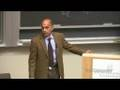1h15m

### 10. Rotations, Part II: Parallel Axis Theorem

For more information about Professor Shankar's book based on the lectures from this course, Fundamentals of Physics: Mechanics, Relativity, and Thermodynamics, visit http://bit.ly/1jFIqNu.

Fundamentals of Physics (PHYS 200)

Part II of Rotations. The lecture begins with an explanation of the Parallel Axis Theorem and how it is applied in problems concerning rotation of rigid bodies. The moment of inertia of a disk is discussed as a demonstration of the theorem. Angular momentum and angular velocity are examined in a variety of problems.

00:00 - Chapter 1. Review and Derive the Parallel Axis Theorem
16:27 - Chapter 2. For System of Masses: Derive KEtotal = ½ MV2 + ½ ICM2
27:55 - Chapter 3. Derive KEtotal in Terms of Equivalent Rotation about Stationary Point
38:40 - Chapter 4. Effect of Rotational Kinetic Energy on Translational Motion for No Skid
43:41 - Chapter 5. Example Problem: Torque on a Disk
49:30 - Chapter 6. Advanced Example Problem: Pulley Rotating and Translating
01:02:141h13m

### 11. Torque

For more information about Professor Shankar's book based on the lectures from this course, Fundamentals of Physics: Mechanics, Relativity, and Thermodynamics, visit http://bit.ly/1jFIqNu.

Fundamentals of Physics (PHYS 200)

This lecture is a continuation of an analogue to Newton's law: ?= l?. While previous problems examined situations in which ? is not zero, this time the focus is on extreme cases in which there is no torque at all. If there is no torque, ? is zero and the angular velocity is constant. The lecture starts with a simple example of a seesaw and moves on to discuss a collection of objects that are somehow subject to a variety of forces but remain in static equilibrium.

00:00 - Chapter 1. Static Equilibrium — Case of Zero-torque, Zero-angular Velocity
03:47 - Chapter 2. The Seesaw Example
12:02 - Chapter 3. The Case of a Rod Supported by Pivot on a Wall
21:04 - Chapter 4. The Case of a Rod Supported by a Wire
29:08 - Chapter 5. The Case of the Leaning Ladder
40:05 - Cha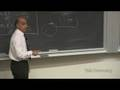1h11m

### 12. Introduction to Relativity

For more information about Professor Shankar's book based on the lectures from this course, Fundamentals of Physics: Mechanics, Relativity, and Thermodynamics, visit http://bit.ly/1jFIqNu.

Fundamentals of Physics (PHYS 200)

This is the first of a series of lectures on relativity. The lecture begins with a historical overview and goes into problems that aim to describe a single event as seen by two independent observers. Maxwell's theory, as well as the Galilean and Lorentz transformations are also discussed.

00:00 - Chapter 1. The Meaning of Relativity
18:10 - Chapter 2. The Galilean Transformation and its Consequences
31:35 - Chapter 3. The Medium of Light
43:22 - Chapter 4. The Two Postulates of Relativity
46:48 - Chapter 5. Length Contraction and Time Dilation
55:34 - Chapter 6. Deriving the Lorentz Transformation

Complete course materials are available at the Yale Online website: online.yale.edu

This course was recorded in Fall 2006.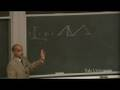1h08m

### 13. Lorentz Transformation

For more information about Professor Shankar's book based on the lectures from this course, Fundamentals of Physics: Mechanics, Relativity, and Thermodynamics, visit http://bit.ly/1jFIqNu.

Fundamentals of Physics (PHYS 200)

This lecture offers detailed analysis of the Lorentz transformations which relate the coordinates of an event in two frames in relative motion. It is shown how length, time and simultaneity are relative.

00:00 - Chapter 1. Describing an Event with Two Observers
33:58 - Chapter 2. The Relativity of Simultaneity
41:57 - Chapter 3. Time Dilation
53:41 - Chapter 4. The Twin Paradox
01:00:02 - Chapter 5. Length Contraction

Complete course materials are available at the Yale Online website: online.yale.edu

This course was recorded in Fall 2006.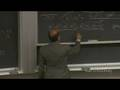1h12m

### 14. Introduction to the Four-Vector

For more information about Professor Shankar's book based on the lectures from this course, Fundamentals of Physics: Mechanics, Relativity, and Thermodynamics, visit http://bit.ly/1jFIqNu.

Fundamentals of Physics (PHYS 200)

The four-vector is introduced that unifies space-time coordinates x, y, z and t into a single entity whose components get mixed up under Lorentz transformations. The length of this four-vector, called the space-time interval, is shown to be invariant (the same for all observers). Likewise energy and momentum are unified into the energy-momentum four-vector.

00:00 - Chapter 1. Recap—Consequences of the Lorentz Transformations
06:25 - Chapter 2. Causality Paradoxes: "Killing the Grandmother"
15:22 - Chapter 3. A New Understanding of Space-Time
25:51 - Chapter 4. Introducing the Fourth Dimension and Four-Vector Algebra
44:09 - Chapter 5. The Space-Time Interval, or "Proper Time"
51:47 - Chapter 6. Deriving the Velocity and Momentum Vectors in Space-Time
01:04:40 - C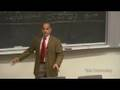1h11m

### 15. Four-Vector in Relativity

For more information about Professor Shankar's book based on the lectures from this course, Fundamentals of Physics: Mechanics, Relativity, and Thermodynamics, visit http://bit.ly/1jFIqNu.

Fundamentals of Physics (PHYS 200)

The discussion of four-vector in relativity continues but this time the focus is on the energy-momentum of a particle. The invariance of the energy-momentum four-vector is due to the fact that rest mass of a particle is invariant under coordinate transformations.

00:00 - Chapter 1. Recap: The Four-Vectors of Position, Velocity and Momentum in Space-Time
15:53 - Chapter 2. The Energy-Momentum Four-Vector
32:20 - Chapter 3. Relativistic Collisions
41:09 - Chapter 4. Law of Conservation of Energy and Momentum Using the Energy-Momentum Four-Vector

Complete course materials are available at the Yale Online website: online.yale.edu

This course was recorded in Fall 2006.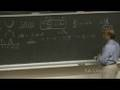1h13m

### 16. The Taylor Series and Other Mathematical Concepts

For more information about Professor Shankar's book based on the lectures from this course, Fundamentals of Physics: Mechanics, Relativity, and Thermodynamics, visit http://bit.ly/1jFIqNu.

Fundamentals of Physics (PHYS 200)

The lecture covers a number of mathematical concepts. The Taylor series is introduced and its properties discussed, supplemented by various examples. Complex numbers are explained in some detail, especially in their polar form. The lecture ends with a discussion of simple harmonic motion.

00:00 - Chapter 1. Derive Taylor Series of a Function, f as [? (0, ?)fnxn/n!]
14:21 - Chapter 2. Examples of Functions with Invalid Taylor Series
17:30 - Chapter 3. Taylor Series for Popular Functions(cos x, ex,etc)
23:31 - Chapter 4. Derive Trigonometric Functions from Exponential Functions
31:41 - Chapter 5. Properties of Complex Numbers
42:40 - Chapter 6. Polar Form of Complex Numbers
50:04 - Chapter 7. Simple Harmonic Motions
01:03:07 - Chapter 8. Law of Conservation of Ene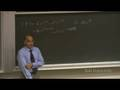1h14m

### 17. Simple Harmonic Motion

For more information about Professor Shankar's book based on the lectures from this course, Fundamentals of Physics: Mechanics, Relativity, and Thermodynamics, visit http://bit.ly/1jFIqNu.

Fundamentals of Physics (PHYS 200)

The focus of the lecture is simple harmonic motion. Professor Shankar gives several examples of physical systems, such as a mass M attached to a spring, and explains what happens when such systems are disturbed. Amplitude, frequency and period of simple harmonic motion are also defined in the course of the lecture. Several problems are solved in order to demonstrate various cases of oscillation.

00:00 - Chapter 1. Example Equations of Oscillating Objects
10:49 - Chapter 2. Superposition of Solutions to Linear (Harmonic) Equations
30:16 - Chapter 3. Conditions for Solutions to Harmonic Equations
38:57 - Chapter 4. Exponential Functions as Generic Solutions
50:48 - Chapter 5. Undamped, Under-damped and Over-damped Oscillations
01:00:28 - Chapter 6. Driving Harmonic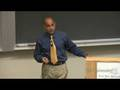1h15m

### 18. Simple Harmonic Motion (cont.) and Introduction to Waves

For more information about Professor Shankar's book based on the lectures from this course, Fundamentals of Physics: Mechanics, Relativity, and Thermodynamics, visit http://bit.ly/1jFIqNu.

Fundamentals of Physics (PHYS 200)

This lecture continues the topic of harmonic motions. Problems are introduced and solved to explore various aspects of oscillation. The second half of the lecture is an introduction to the nature and behavior of waves. Both longitudinal and transverse waves are defined and explained.

00:00 - Chapter 1. Free Vibration: Oscillation Under F=0
08:20 - Chapter 2. Initial Conditions at Start of Oscillation
18:52 - Chapter 3. Solution to Harmonic Equation under Driving Force
30:01 - Chapter 4. Properties of the Oscillating Function, Resonance
39:23 - Chapter 5. Complete Solution = Complimentary + Particular Solutions
43:40 - Chapter 6. Introduction to Longitudinal and Transverse Waves
52:55 - Chapter 7. Derive Wave Equation as Differential Equation
01:04:40 - Chapter 8.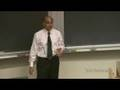1h11m

### 19. Waves

For more information about Professor Shankar's book based on the lectures from this course, Fundamentals of Physics: Mechanics, Relativity, and Thermodynamics, visit http://bit.ly/1jFIqNu.

Fundamentals of Physics (PHYS 200)

Waves are discussed in further detail. Basic properties of the waves such as velocity, energy, intensity, and frequency are discussed through a variety of examples. The second half of the lecture deals specifically with superposition of waves. Constructive and destructive interferences are defined and discussed.

00:00 - Chapter 1. General Solution of Wave Equation
08:51 - Chapter 2. Spatial and Temporal Periodicity: Frequency, Period
17:39 - Chapter 3. Wave Energy and Power Transmitted
30:02 - Chapter 4. Doppler Effect
38:58 - Chapter 5. Superposition of Waves
48:57 - Chapter 6. Constructive and Destructive Interference, Double Slit Experiment
01:01:30 - Chapter 7. Modes of Vibration: Application to Musical Instruments

Complete course materials are available at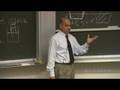1h12m

### 20. Fluid Dynamics and Statics and Bernoulli's Equation

For more information about Professor Shankar's book based on the lectures from this course, Fundamentals of Physics: Mechanics, Relativity, and Thermodynamics, visit http://bit.ly/1jFIqNu.

Fundamentals of Physics (PHYS 200)

The focus of the lecture is on fluid dynamics and statics. Different properties are discussed, such as density and pressure. Archimedes' Principle is introduced and demonstrated through a number of problems. The final topic of the lecture is Bernoulli's Equation.

00:00 - Chapter 1. Introduction to Fluid Dynamics and Statics — The Notion of Pressure
04:14 - Chapter 2. Fluid Pressure as a Function of Height
20:49 - Chapter 3. The Hydraulic Press
26:32 - Chapter 4. Archimedes' Principle
36:36 - Chapter 5. Bernoulli's Equation
39:12 - Chapter 6. The Equation of Continuity
53:41 - Chapter 7. Applications of Bernoulli's Equation

Complete course materials are available at the Yale Online website: online.yale.edu

This course was recorded in Fall 2006.